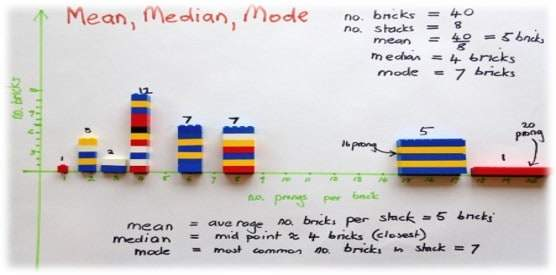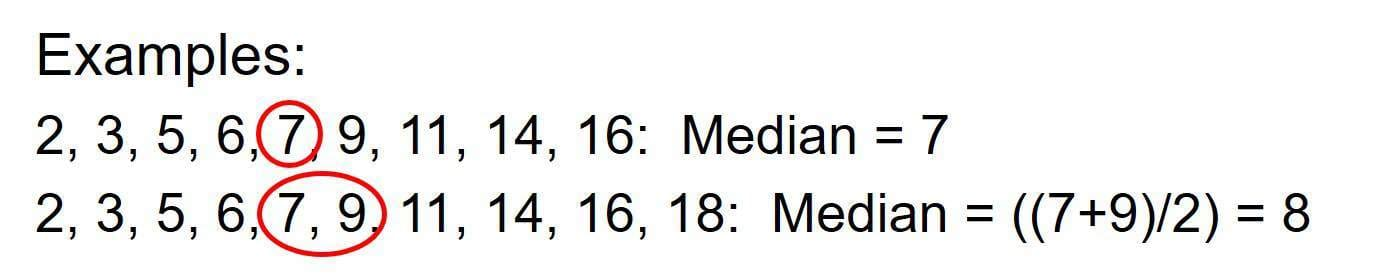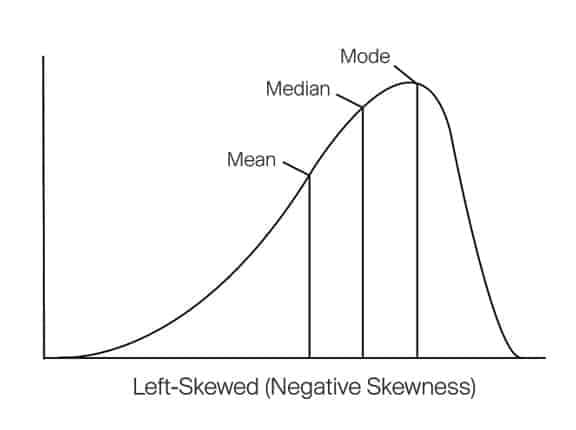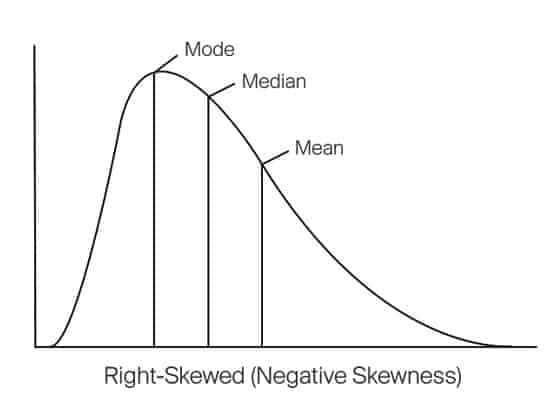Select Page## Using “Median” as a Measures of Central Tendency

A measure of central tendency is a single value that describes the way in which a set of data cluster around a central value. In other words, it is a way to describe the center of a data set.

The three main measures of central tendency are:

### In this article we will discuss the Median of the Distribution

The median is the literal middle of the data set, when arranged in order from smallest to largest. For an odd sized sample, the median is equal to the literal middle number. To find the median an even sized sample, add the two middle numbers and then divide the result by two.The median of a distribution of data is determined as the 50th percentile point once the distribution has been defined.

For normal distributions, the median value occurs at or close to the mean of the distribution. This is also where the mode, or high point (most frequently occurring value), of the distribution occurs.

For distributions that are skewed to the left, both the median and mode occur to the left of (are smaller than) the average of the distribution.For distributions that are skewed to the right, both the median and mode occur to the right of (are larger than) the average of the distribution.### Finding skewed distributions in a Lean Six Sigma project?

I normally find skewed distributions in data representing time. Foe example, a all centers time to resolve a level one help desk ticket. The call center is continuously trying to decrease the time to resolve a level one help desk ticket. The data set should show that most of the data should cluster closer to zero. This would represent skewed data that would have a central tendency best described by the median.LEARN THE 10 SIMPLE STEPS THAT WE TEACH LEAN SIX SIGMA GREEN BELTS TO GET AMAZING RESULTS FROM THEIR PROJECTS.